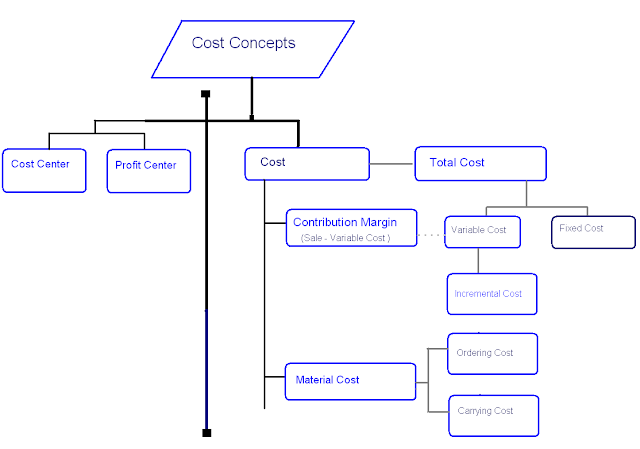222

# Why Accounting Education Important# Why Accounting Education Volunteer## Become Volunteer Teacher

In cost accounting, there are some cost concepts which you should know for understanding the science of cost accounting perfectly. Today, we are explaining all these cost concepts.

1. Cost

Cost is monetary consideration for getting stock, asset or service. It is expense for buying goods or asset or service. In simple word, cost is the value of sacrifice for getting something. For production and sale, all paid and payable expenses will include in cost. Concept of cost will be different when our aim will different. If we have to calculate the cost of sale, we have to calculate total cost. When we have to calculate the cost of stock, we need to calculate the cost of production.

2. Cost Center

Cost center is a small department or branch or division which is helpful for calculating the cost and control over costs. For example for production of any product need to process in 6 machines. All these 6 machines will become cost center because we can calculate the cost of processing in each machine. We can make the responsible to the manager of each cost center.

3. Profit Center

Profit center is that part of business organisation in which we calculate the net profit. Every person who is the  incharge of profit center will be responsible both incomes and expenses of his profit center.

4. Conversion Cost

Conversion cost is the part of cost concept. Conversion cost is the cost to convert raw material into finished stock. We can calculate conversion cost by deducting direct material cost  from factory cost or we can calculate it by adding direct labor cost with factory overheads.

5. Contribution Margin

Contribution margin is the difference between sale value and variable cost. We can calculate the % of contribution margin by dividing with sales and multiply with 100.

6. Carrying Cost

Carrying cost is paid for caring the stock. In this cost, we include the rent of stock, cost of capital which is invested in stock, obsolescence of stock cost and other store expenses.

7. Out of Stock Cost

We have to pay or suffer  this cost when there is no goods in our stock. At that time, we have to lose our sale and customer. Our employee will enjoy the idle time. We will suffer the cost of loss of goodwill.

8. Ordering Cost

Ordering cost is the cost which we have to pay for placing new purchasing order.

9. Development Cost

Development cost is the cost which is paid for developing a new product from constructional process.

10. Policy Cost

Policy cost is the cost which is incurred for implementation of the policies of company.

11. Discretionary Cost

Discretionary cost is the cost which can be reduced by manager's good decisions. So, it is called managed cost. For example, advertising cost is discretionary cost. Manager will check whether the advertising has increased the return or sale. If not, manager can low this budget or postpone this cost.

12. Idle Facilities Cost

If employees has not stock or some equipment or machines are not repaired, at that time, employees will be idle. But we have to pay the wage and salary to them. So, this cost will become idle facilities cost.

13. Expired Cost

This is one of the important cost concept. It means the expenses which were paid for getting benefits. If we got all benefits, same expenses will become expired cost. For example, we have used the car for our business in past 10 years. After deducting depreciation, it is scrape. So, cost of car which we have used in 10 years will be expired cost.

14. Incremental Cost

Incremental cost is the cost for increasing one more unit of production. It is also called marginal cost.

Added Value is increase in the cost of product due to changing the product by production process or bring quality by improving the product. In simple word, Value added is difference between sale price and cost price of any product. We are earning profit because we have added some value in it. For example, a retailer buys the goods from city and sell it in village. By doing hard work and carrying goods to village is sufficient for getting earning from its sale.

16. Urgent Cost

Urgent cost is the cost which are incurred for stopping the disturbance or delay in the production.

17. Postponable Cost

Postponable cost are those expenses which can easily be transferred to future time. For example, advertising cost may be postponable cost because we can shift it in future date.Name

ltr
item
Accounting Education: Cost Concepts
Cost Concepts
http://2.bp.blogspot.com/-2-nyflVZwkg/Ucv-b15e9TI/AAAAAAAALqc/cAWoUYQQego/s640/cost+concepts.PNG
http://2.bp.blogspot.com/-2-nyflVZwkg/Ucv-b15e9TI/AAAAAAAALqc/cAWoUYQQego/s72-c/cost+concepts.PNG
Accounting Education
http://www.svtuition.org/2013/06/cost-concepts.html
http://www.svtuition.org/
http://www.svtuition.org/
http://www.svtuition.org/2013/06/cost-concepts.html
true
2410664366776677676
UTF-8